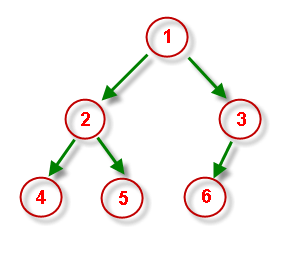Geeks With Blogs
.NET Corner Jeans, .NET and Physics (eka The Quantum Boy)

Well the "brain-brush" series continues. One of my favourite binary tree question would be given a preorder and inorder traversal strings write a function to build the tree. Most of the rubbish text books provide non-programmatic solution to this and rest append it as a to-do. Today I will proceed step-by-step to solve this and that too with same ingredients - delegate, generics and C#2.0. Disclaimer still holds: "This is just a fundamental recapitulation of binary tree and data structure as a whole. Geeks excuse me please for this series.

In the earlier post I discussed the simple data structure of treenode and binary tree. Just to repeat once following is the skeleton of my binary tree class

public class BinaryTree
{
//root node
public TreeNode root;

//Algorithms...InOrder etc.
}

Now for simplicity lets assume that the binary treenode contains single character data. So instead of embedding our tree-building algo inside this generic BinaryTree class I specialized ot to create a subclass called CharBinaryTree. Lets construct the base logic Assume we have following binary tree -Preorder traversal string and Inorder traversal strings are  124536 and 425163 respectively Lets now back calculate and see how one might can possibly build the tree. Firstly preorder string always helps to get the root right. VLR. Does it ring a bell? But then how to differntiate between left subtree(LT) and right subtree(RT). Well simple. Split the inorder string based on the pivotal root. Left substring is LT and right substring is RT. Now we have to recurse. That's fine. But what are the pivotal indexes for LT and RT. Simple - if you can see dry-run the first recursion frame

root := 1, LT := 425, RT := 63
pivot(LT) := pivot+1, pivot(RT) := pivot + len(LT) + 1.

Naturally these pivotal characters will be the roots of the respective subtrees. Enough said. Let's dump the C# code right now.

private TreeNode MakeTree(string inorderString, int preOrderIndex)
{
TreeNode p = new TreeNode(' ');

// terminating condition
if (inorderString.Length == 1)
{
char.TryParse(inorderString, out p.nodeData);
}
else
{
char pivot = this.preorderString[preOrderIndex];
int rootIndexInorder = inorderString.IndexOf(pivot);
string left  = "";
string right = "";

if (rootIndexInorder > 0)
{
left = inorderString.Substring(0, rootIndexInorder);
right = inorderString.Substring(rootIndexInorder + 1);

p.nodeData = pivot;

if (left.Length != 0)
p.left = MakeTree(left, preOrderIndex + 1);

if (right.Length != 0)
p.right = MakeTree(right, preOrderIndex + left.Length + 1);

}

}

return p;
}

This goes the private "workhorse" function as described in my earlier post of the DS-BrainBrush series. Lets see the driver function

public TreeNode MakeTree(string inorderString, string preorderString)
{
//Couple of pre-checks
if ((inorderString.Length == 0) ||
(preorderString.Length == 0))
{
throw new ArgumentException("Traversal strings shouldn't be empty");
}

if (inorderString.Length != preorderString.Length)
{
throw new ArgumentException("Traversal strings should have identical length");
}

//Make a copy of traversal strings
this.preorderString = preorderString;
this.inorderString = inorderString;

//Make a call to "workhorse"
this.root = this.MakeTree(this.inorderString, 0);

return this.root;
}

Everything fits nicely in the "workhorse-driver" model. In the next post of this series I will show how to create a special binary tree called Binary Expression Tree(BET) heavily used in world of lexical analyzer, parser and compilers. See you soon.

Related Posts on Geeks With Blogs Matching Categories

Comments on this post: Binary Tree, C# and Delegates - Part 2

# re: Binary Tree, C# and Delegates - Part 2One smartypants pointed out that sure, this tree can have cycles -- if you have another binary tree implementation that has cycles and then paste such a cyclic tree in. True; what I meant was that using only the stated implementation, it's impossible to create a tree with cycles. If you are hell bent on making a bad tree, you can surely do that. Again, in a future post we'll have a tree which is built up by calling tree "mutating" operations, and those really will be guaranteed acyclic even in a world where there are other bad implementations hanging around.
Left by slots online on Jul 25, 2009 5:32 AM

# re: Binary Tree, C# and Delegates - Part 2I need to implement binary tree in my web application vs 2005 with C#.
i hv to show binary tree according to the data dynamically.

how to procede don konw .. i m nt getting any clue. plz if possible help me as soon as possible.
Left by Deepak on Jan 12, 2010 3:45 AM